# Calculating Heat of Reaction from Heat of Formation

• Calculate standard heat of reaction using standard heats of formation.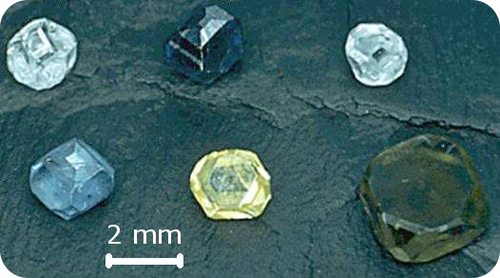Natural diamonds are mined from sites around the world. However, the price of natural diamonds is carefully controlled, so other sources for diamonds are being explored. Several different methods for producing synthetic diamonds are available, usually involving treating carbon at very high temperatures and pressures. The diamonds produced are now of high quality, but are primarily used in industrial applications. Diamonds are one of the hardest materials available and are widely used for cutting and grinding tools.

### Calculating Heat of Reaction from Heat of Formation

An application of Hess’s law allows us to use standard heats of formation to indirectly calculate the heat of reaction for any reaction that occurs at standard conditions. An enthalpy change that occurs specifically under standard conditions is called the standard enthalpy (or heat) of reaction and is given the symbol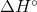. The standard heat of reaction can be calculated by using the following equation.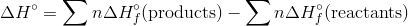The symbol Σ is the Greek letter sigma and means “the sum of”. The standard heat of reaction is equal to the sum of all the standard heats of formation of the products minus the sum of all the standard heats of formation of the reactants. The symbol “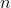” signifies that each heat of formation must first be multiplied by its coefficient in the balanced equation.

 Substance ΔHfº (kJ/mol) Substance ΔHfº (kJ/mol) Al2O3(s) -1669.8 H2O2(l) -187.6 BaCl2(s) -860.1 KCl(s) -435.87 Br2(g) 30.91 NH3(g) -46.3 C (s, graphite) 0 NO(g) 90.4 C (s, diamond) 1.90 NO2(g) 33.85 CH4(g) -74.85 NaCl -411.0 C2H5OH(l) -276.98 O3(g) 142.2 CO(g) -110.5 P(s, white) 0 CO2(g) -393.5 P(s, red) -18.4 CaO(s) -635.6 PbO(s) -217.86 CaCO3(s) -1206.9 S(rhombic) 0 HCl(g) -92.3 S(monoclinic) 0.30 CuO(s) -155.2 SO2(g) -296.1 CuSO4(s) -769.86 SO3(g) -395.2 Fe2O3(s) -822.2 H2S(g) -20.15 H2O(g) -241.8 SiO2 -859.3 H2O(l) -285.8 ZnCl2 -415.89

#### Sample Problem: Calculating Standard Heat of Reaction

Calculate the standard heat of reaction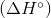for the reaction of nitrogen monoxide gas with oxygen to form nitrogen dioxide gas.

Step 1: List the known quantities and plan the problem .

Known

•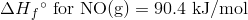•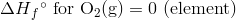•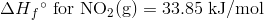Unknown

•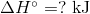First write the balanced equation for the reaction. Then apply the equation to calculate the standard heat of reaction for the standard heats of formation.

Step 2: Solve .

The balanced equation is: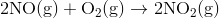Applying the equation form the text: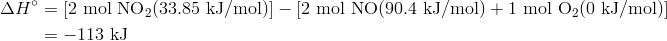The standard heat of reaction is -113 kJ.

The reaction is exothermic, which makes sense because it is a combustion reaction and combustion reactions always release heat.

#### Summary

• Standard heats of reaction can be calculated from standard heats of formation.

#### Practice

Do the practice exercises at the link below:

http://wps.prenhall.com/wps/media/objects/3311/3390593/blb0507.html

#### Review

Questions

1. Is a Hess’ Law calculation a direct determination of a standard heat of reaction?
2. What is the purpose of thein the equation?
3. What does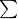stand for?
• standard heat of reaction: An enthalpy change that occurs specifically under standard conditions.